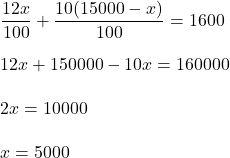## Loans Bill took out two loans that totaled $15,000. One of the loans charges 12% per year, and the other charges 10% Question Loans Bill took out two loans that totaled$15,000. One

of the loans charges 12% per year, and the other charges

10% per year. If the total interest charged in the first year

is $1,600, how much was each loan? in progress 0 5 months 2021-08-13T22:20:07+00:00 1 Answers 1 views 0 ## Answers ( ) 1. Let, money in loan with 12% interest is x. So, money in loan with 10% interest is 15000-x. Now , sum of interest :Therefore, money on 12% interest is$5000 and 10% interest is \$10000.

Hence, this is the required solution.